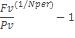## Excel Office

Excel How Tos, Tutorials, Tips & Tricks, Shortcuts

# RRI function: Description, Usage, Syntax, Examples and Explanation

## What is RRI function in Excel?

RRI function is one of the Financial functions in Microsoft Excel that returns an equivalent interest rate for the growth of an investment.

## Syntax of RRI function

RRI(nper, pv, fv)

The RRI function syntax has the following arguments.

• Nper    Required. Nper is the number of periods for the investment.
• Pv    Required. Pv is the present value of the investment.
• Fv    Required. Fv is the future value of the investment.

RRI returns the interest rate given nper (the number of periods), pv (present value), and fv (future value), calculated by using the following equation:## RRI formula explanation

• If argument values are not valid, RRI returns the #NUM! error value.
• If arguments are not using valid data types, RRI returns the #VALUE! error value.

## Example of RRI function

Steps to follow:

1. Open a new Excel worksheet.

2. Copy data in the following table below and paste it in cell A1

Note: For formulas to show results, select them, press F2 key on your keyboard and then press Enter.

You can adjust the column widths to see all the data, if need be.

 Formula Description Result =RRI(96,10000,11000) Returns an equivalent interest rate for the growth of an investment of \$10,000 with a future value of \$11,000, for 8 years (0.012, or 1.2%). 0.0009933